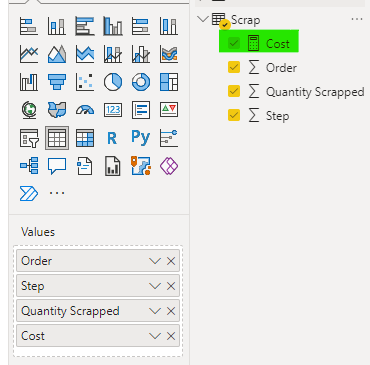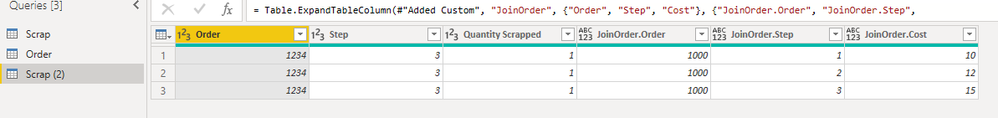cancel
Showing results for
Did you mean:Frequent Visitor

## Join with Clauses

Hi,

I am looking for a way to merge two tables adding some clauses to the join.

For example:

Scrap Table:

 Order Step Quantity Scrapped Cost 1234 3 1 37

Order table:

 Order Step Cost 1000 1 10 1000 2 12 1000 3 15 1000 4 10 1000 5 10

The COST column from the Scrap table is the sum of all Cost column from Order table which step column is <= step column from scrap table.

Using SQL it would be more or less like below:

Select Scrap.order, Scrap.step , Scrap.QuantityScrapped , Sum(Order.Cost)

From Scrap Join Order

ON Scrap.order = Order.order

AND Order.step <= Scrap.Step

1 ACCEPTED SOLUTIONCommunity Support

Hi @Diogo_Dalla ,

Please try the following measure or custom column(PQ).

Measure:

``````SumCost =
SUMX (
SUMMARIZE (
Scrap,
Scrap[Order],
"_sum",
CALCULATE (
SUM ( 'Order'[Cost] ),
FILTER (
'Order',
'Order'[Step] <= MAX ( Scrap[Step] )
&& 'Order'[Order] = MAX ( 'Scrap'[Order] )
)
)
),
[_sum]
)``````PQ Column:

``````JoinOrderCost =
let
myfunction = (CurrentOrder, CurrentStep) =>
let
SelectRows = Table.SelectRows(Order, each [Order] = CurrentOrder and [Step] <= CurrentStep)
in
SelectRows,
Data = List.Sum( myfunction([Order],[Step])[Cost])
in
Data``````If the problem is still not resolved, please provide detailed error information or the expected result you expect. Let me know immediately, looking forward to your reply.
Best Regards,
Winniz
If this post helps, then please consider Accept it as the solution to help the other members find it more quickly.

7 REPLIES 7Super User

Hi,

Would you be OK with a DAX solution?

Regards,
Ashish Mathur
http://www.ashishmathur.comCommunity Support

Hi @Diogo_Dalla ,

``````Cost =
CALCULATE (
SUM ( 'Order'[Cost] ),
FILTER ( 'Order', 'Order'[Step] <= MAX ( Scrap[Step] ) )
)``````If the problem is still not resolved, please provide detailed error information or the expected result you expect. Let me know immediately, looking forward to your reply.
Best Regards,
Winniz
If this post helps, then please consider Accept it as the solution to help the other members find it more quickly.Frequent Visitor

Hi,

I checked the model that you sent, but I didn't find where you have added the DAX Code.

Have you merged the two tables?

Thank you,

Diogo DallaCommunity Support

Hi @Diogo_Dalla ,

I did not merge the two tables, I simply created a measure as shown below.Or try to create a custom column in Power Query to get the data from the Order table and extend it.

``= Table.SelectRows(Order, each ([Step] <= #"Changed Type"{0}[Step]))``If the problem is still not resolved, please provide detailed error information or the expected result you expect. Let me know immediately, looking forward to your reply.
Best Regards,
Winniz
If this post helps, then please consider Accept it as the solution to help the other members find it more quickly.Frequent Visitor

Hi,

I think that it is almost there.

Doing the models that you shared I found the following:

*  Model1 - The DAX code below is not filtering by Order.

``FILTER ( 'Order', 'Order'[Step] <= MAX ( Scrap[Step] ) )``

``````Cost =
CALCULATE (
SUM ( 'Order'[Cost] ),
FILTER ( 'Order', 'Order'[Step] <= MAX ( Scrap[Step] ) && 'order'[order] = Max(Scrap[order]) )
)``````

But it will bring the Max Scrap.Step and the Max Scrap.order

I was able to do it in Excel with power query this way:

``````SELECT  `'SCRAP\$'`.order
, `'SCRAPa\$'`.Step
, `'SCRAP\$'`.Quantity
, (SELECT SUM(`'ORDER\$'`.Cost) FROM `'ORDER\$'` `'ORDER\$'`
WHERE `'ORDER\$'`.order = `'SCRAP\$'`.Order
and `'ORDER\$'`.Step <= `'Scrap\$'`.Sterp)
FROM  `'SCRAP\$'` `'SCRAP\$'```````

It merged the two queries in one bringing the total cost from Order table.

* Model2 - Will not bring the sum of the costs from order table.

Thank you,

Diogo DallaCommunity Support

Hi @Diogo_Dalla ,

Please try the following measure or custom column(PQ).

Measure:

``````SumCost =
SUMX (
SUMMARIZE (
Scrap,
Scrap[Order],
"_sum",
CALCULATE (
SUM ( 'Order'[Cost] ),
FILTER (
'Order',
'Order'[Step] <= MAX ( Scrap[Step] )
&& 'Order'[Order] = MAX ( 'Scrap'[Order] )
)
)
),
[_sum]
)``````PQ Column:

``````JoinOrderCost =
let
myfunction = (CurrentOrder, CurrentStep) =>
let
SelectRows = Table.SelectRows(Order, each [Order] = CurrentOrder and [Step] <= CurrentStep)
in
SelectRows,
Data = List.Sum( myfunction([Order],[Step])[Cost])
in
Data``````If the problem is still not resolved, please provide detailed error information or the expected result you expect. Let me know immediately, looking forward to your reply.
Best Regards,
Winniz
If this post helps, then please consider Accept it as the solution to help the other members find it more quickly.Impactful Individual

https://www.ehansalytics.com/blog/2020/9/8/conditional-merge-in-power-query

Amira Bedhiafi
Full Stack Business Intelligence Consultant @Capgemini